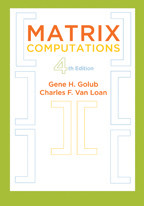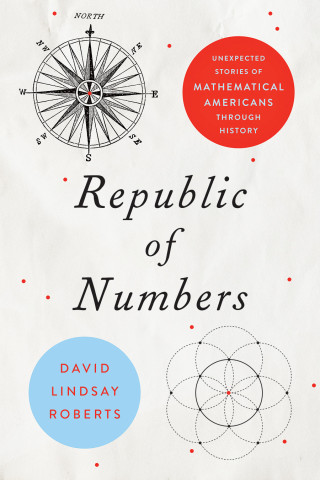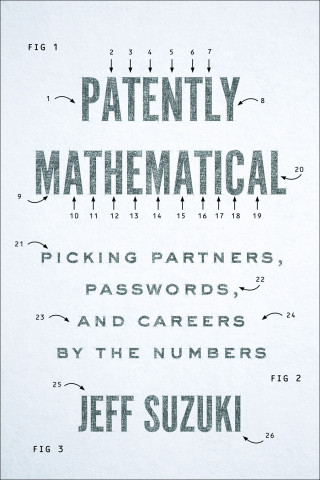Back to Results# Calculus in Context

Background, Basics, and Applications

Alexander J. Hahn

Publication Date
Request Exam CopyRequest Review Copy

A new approach to teaching calculus that uses historical examples and draws on applications from science and engineering.

Breaking the mold of existing calculus textbooks, Calculus in Context draws students into the subject in two new ways. Part I develops the mathematical preliminaries (including geometry, trigonometry, algebra, and coordinate geometry) within the historical frame of the ancient Greeks and the heliocentric revolution in astronomy. Part II starts with comprehensive and modern treatments of the fundamentals of both differential and integral calculus, then turns to a wide-ranging…

A new approach to teaching calculus that uses historical examples and draws on applications from science and engineering.

Breaking the mold of existing calculus textbooks, Calculus in Context draws students into the subject in two new ways. Part I develops the mathematical preliminaries (including geometry, trigonometry, algebra, and coordinate geometry) within the historical frame of the ancient Greeks and the heliocentric revolution in astronomy. Part II starts with comprehensive and modern treatments of the fundamentals of both differential and integral calculus, then turns to a wide-ranging discussion of applications. Students will learn that core ideas of calculus are central to concepts such as acceleration, force, momentum, torque, inertia, and the properties of lenses.

Classroom-tested at Notre Dame University, this textbook is suitable for students of wide-ranging backgrounds because it engages its subject at several levels and offers ample and flexible problem set options for instructors. Parts I and II are both supplemented by expansive Problems and Projects segments. Topics covered in the book include:

• the basics of geometry, trigonometry, algebra, and coordinate geometry and the historical, scientific agenda that drove their development
• a brief, introductory calculus from the works of Newton and Leibniz
• a modern development of the essentials of differential and integral calculus
• the analysis of specific, relatable applications, such as the arc of the George Washington Bridge; the dome of the Pantheon; the optics of a telescope; the dynamics of a bullet; the geometry of the pseudosphere; the motion of a planet in orbit; and the momentum of an object in free fall.

Calculus in Context is a compelling exploration—for students and instructors alike—of a discipline that is both rich in conceptual beauty and broad in its applied relevance.

Calculus in Context

Alexander J. Hahn

Publication Date
Request Exam CopyRequest Review Copy
Related

## Related BooksMatrix Computations

Gene H. Golub and Charles F. Van Loan

fourth edition
\$76.00
Matrix Computations

Gene H. Golub and Charles F. Van Loan

fourth edition
Publication DateSupermath

Anna Weltman

\$24.95
Supermath

Anna Weltman

Publication DateRepublic of Numbers

David Lindsay Roberts

\$29.95
Republic of Numbers

David Lindsay Roberts

Publication DatePatently Mathematical

Jeff Suzuki

\$37.00
Patently Mathematical

Jeff Suzuki

Publication Date
Reviews

## Reviews

The depth of detail in each application [offered by Calculus in Context] provides an excellent structure for guiding students through the "why should we care" moments that every calculus class experiences.

Recommended.

Hahn's book is the perfect choice for college and university teachers who want to teach calculus with reference to its origins and applications.

Very well written in an engaging and enthusiastic style: it is very suitable for first year students, is perhaps not too demanding for students about to enter university, and it is particularly useful to those with more than a passing interest in astronomy. There is plenty to learn for the reader, and the massive text is also a good reference book on calculus. This labour of love from the author more than satisfies the high hopes for a good calculus book... and I highly recommend it.

## Book Details

Publication Date
Status
Available
Trim Size
7
x
10
Pages
712
ISBN
9781421422305
Illustration Description
645 line drawings
Subject

Preface
Part I
1. The Astronomy and Geometry of the Greeks
1.1. The Greeks Explain the Universe
1.2. Achieving the Impossible?
1.3. Greek Geometry
1.4. The Pythagorean Theorem
1.5. The Radian Measure of an

Preface
Part I
1. The Astronomy and Geometry of the Greeks
1.1. The Greeks Explain the Universe
1.2. Achieving the Impossible?
1.3. Greek Geometry
1.4. The Pythagorean Theorem
1.5. The Radian Measure of an Angle
1.6. Greek Trigonometry
1.7. Aristarchus Sizes Up the Universe
1.8. Problems and Projects
2. The Genius of Archimedes
2.1. The Conic Sections
2.2. The Question of Area
2.3. Playing with Squares
2.4. The Area of a Parabolic Section
2.5. The Method of Archimedes
2.6. Problems and Projects
3. A New Astronomy
3.1. A Fixed Sun at the Center
3.2. Copernicus's Model of Earth's Orbit
3.3. About the Distances of the Planets from the Sun
3.4. Tycho Brahe and Parallax
3.5. Kepler's Elliptical Orbits
3.6. The Studies of Galileo
3.7. The Size of the Solar System
3.8. Problems and Projects
4. The Coordinate Geometry of Descartes
4.1. The Real Numbers
4.2. The Coordinate Plane
4.3. About the Parabola
4.4. About the Ellipse
4.5. Quadratic Equations in x and y
4.6. Circles and Trigonometry
4.7. Problems and Projects
5. The Calculus of Leibniz
5.1. Straight Lines
5.2. Tangent Lines to Curves
5.3. The Function Concept
5.4. The Derivative of a Function
5.5. Fermat, Kepler, and Wine Barrels
5.6. The Definite Integral
5.7. Cavalieri's Principle
5.8. Differentials and the Fundamental Theorem
5.9. Volumes of Revolution
5.10. Problems and Projects
6. The Calculus of Newton
6.1. Simple Functions and Areas
6.2. The Derivative of a Simple Function
6.3. From Simple Functions to Power Series
6.4. The Mathematics of a Moving Point
6.5. Galileo and Acceleration
6.6. Dealing with Forces
6.7. The Trajectory of a Projectile
6.8. Newton Studies the Motion of the Planets
6.9. Connecting Force and Geometry
6.10. The Law of Universal Gravitation
6.11. Problems and Projects
Part II
7. Differential Calculus
7.1. Mathematical Functions
7.2. A Study of Limits
7.3. Continuous Functions
7.4. Differentiable Functions
7.5. Computing Derivatives
7.6. Some Theoretical Concerns
7.7. Derivatives of Trigonometric Functions
7.8. Understanding Functions
7.9. Graphing Functions
7.10. Exponential Functions
7.11. Logarithm Functions
7.12. Hyperbolic Functions
7.14. Problems and Projects
8. Applications of Differential Calculus
8.1. Derivatives as Rates of Change
8.1.1. Growth of Organisms
8.1.3. Cost of Production
8.2. The Pulley Problem of L'Hospital
8.2.1. The Solution Using Calculus
8.2.2. The Solution by Balancing Forces
8.3. The Suspension Bridge
8.4. An Experiment of Galileo
8.4.1. Sliding Ice Cubes and Spinning Wheels
8.4.2. Torque and Rotational Inertia
8.4.3. The Mathematics behind Galileo's Experiment
8.5. From Fermat's Principle to the Reflecting Telescope
8.5.1. Fermat's Principle and the Reflection of Light
8.5.2. The Refraction of Light
8.5.4. Refracting and Reflecting Telescopes
8.6. Problems and Projects
9. The Basics of Integral Calculus
9.1. The Definite Integral of a Function
9.2. Volume and the Definite Integral
9.3. Lengths of Curves and the Definite Integral
9.4. Surface Area and the Definite Integral
9.5. The Definite Integral and the Fundamental Theorem
9.6. Area as Antiderivative
9.7. Finding Antiderivatives
9.7.1. Integration by Substitution
9.7.2. Integration by Parts
9.7.3. Some Algebraic Moves
9.8. Inverse Functions
9.9. Inverse Trigonometric and Hyperbolic Functions
9.9.1. Trigonometric Inverses
9.9.2. Hyperbolic Inverses
9.10. Trigonometric and Hyperbolic Substitutions
9.11. Some Integral Formulas
9.12. The Trapezoidal and Simpson Rules
9.13. One Loop of the Sine Curve
9.14. Problems and Projects
10. Applications of Integral Calculus
10.1. Estimating the Weight of Domes
10.1.1. The Hagia Sophia
10.1.2. The Roman Pantheon
10.2. The Cables of a Suspension Bridge
10.3. From Pocket Watch to Pseudosphere
10.3.1. Volume and Surface Area of Revolution of the Tractrix
10.3.2. The Pseudosphere
10.4. Calculating the Motion of a Planet
10.4.1. Determining Position in Terms of Time
10.4.2. Determining Speed and Direction
10.4.3. Earth, Jupiter, and Halley
10.5. Integral Calculus and the Action of Forces
10.5.1. Work and Energy, Impulse and Momentum
10.5.2. Analysis of Springs
10.5.3. The Force in a Gun Barrel
10.5.4. The Springfield Rifle
10.6. Problems and Projects
11. Basics of Differential Equations
11.1. First-Order Separable Differential Equations
11.2. The Method of Integrating Factors
11.3. Direction Fields and Euler's Method
11.4. The Polar Coordinate System
11.5. The Complex Plane
11.6. Second-Order Differential Equations
11.7. The Basics of Power Series
11.8. Taylor and Maclaurin Series
11.9. Solving a Second-Order Differential Equation
11.10. Free Fall with Air Resistance
11.10.1. Going Up
11.10.2. Coming Down
11.10.3. Bullets and Ping-Pong Balls
11.11. Systems with Springs and Damping Elements
11.11.1. The Family Sedan and the Stock Car
11.12. More about Hanging Cables
11.13. Problems and Projects
12. Polar Calculus and Newton's Planetary Orbits
12.1. Graphing Polar Equations
12.2. The Conic Sections in Polar Coordinates
12.3. The Derivative of a Polar Function
12.4. The Lengths of Polar Curves
12.5. Areas in Polar Coordinates
12.6. Equiangular Spirals
12.7. Centripetal Force in Cartesian Coordinates
12.8. Going Polar
12.9. From Conic Section to Inverse Square Law and Back Again
12.10. Gravity and Geometry
12.11. Spiral Galaxies
12.12. Problems and Projects
References
Image Credits and Notes
Index

Author BioFeatured Contributor

## Alexander J. Hahn

Alexander J. Hahn is a professor of mathematics at the University of Notre Dame. He is the author of Basic Calculus: From Archimedes to Newton to Its Role in Science and Mathematical Excursions to the World’s Great Buildings.
Resources